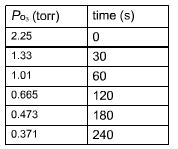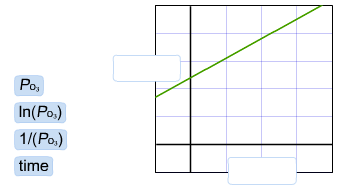# Problem: The reaction between carbon disulfide and ozone described by the equation CS2(g) + 2O3(g) → CO2(g) + 2SO2(g) was studied using a large excess of CS 2(g). The pressure of ozone as a function of time is given in the table. What is the order of this reaction with respect to ozone? a. zerob. firstc. secondThe graph is derived from the data in the table. Label the axes of the graph correctly such that the slope of the line is equal to the rate constant.

###### FREE Expert Solution

The order of a chemical reaction is defined as the sum of power of concentrations of the reactants in the rate equation.

Order of a chemical reaction is determined experimentally; it cannot be determined using stoichiometry from balanced chemical equation.###### Problem Details

The reaction between carbon disulfide and ozone described by the equation

CS2(g) + 2O3(g) → CO2(g) + 2SO2(g)

was studied using a large excess of CS 2(g). The pressure of ozone as a function of time is given in the table.What is the order of this reaction with respect to ozone?

a. zero

b. first

c. second

The graph is derived from the data in the table. Label the axes of the graph correctly such that the slope of the line is equal to the rate constant.